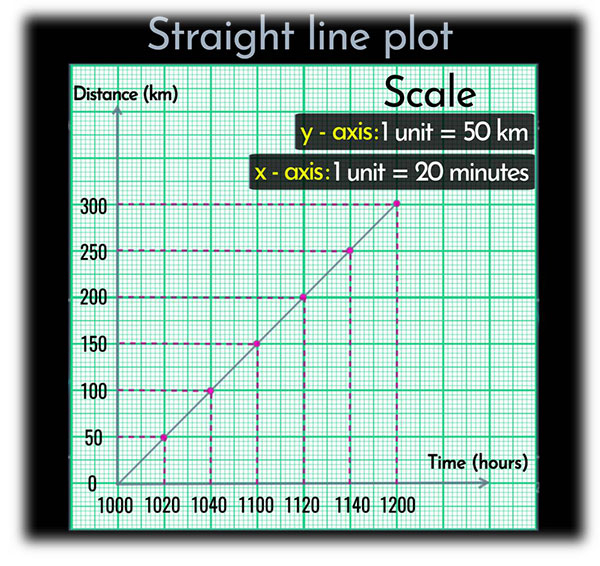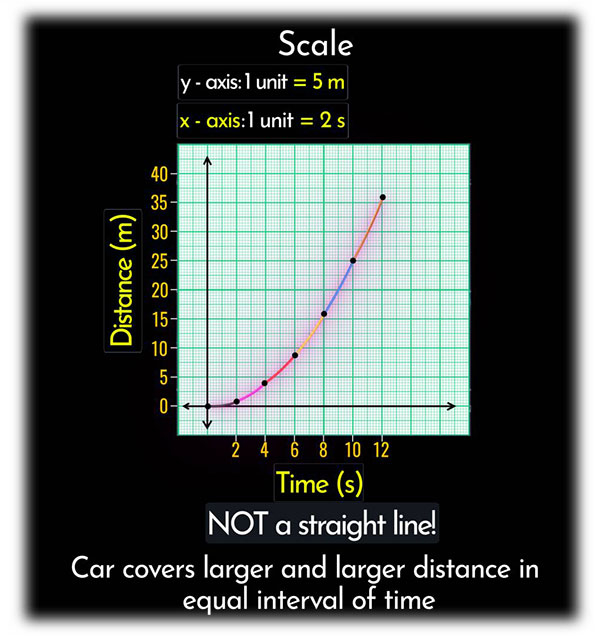# What Is Nature Of Distance -time Graphs For Uniform And Non-Unifrom Motion Of An Object?

Uniform motion is a motion in which a body travels the equal distance in equal intervals of time. The nature of the distance-time graph for uniform motion in a straight line as shown below.But a non-uniform motion is a motion in which a body covers unequal distances in equal intervals of time. It is a curved path as shown below.Updated on: 10-Oct-2022

31 Views